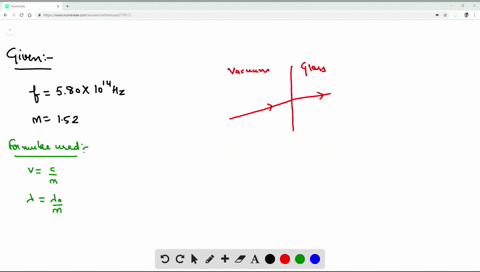Enroll in one of our FREE online STEM summer camps. Space is limited so join now!View Summer Courses### Light with a frequency of $5.80 \times 10^{14}$ H…

01:52Wesleyan University

Need more help? Fill out this quick form to get professional live tutoring.

Get live tutoring
Problem 3

A beam of light has a wavelength of 650 nm in vacuum. (a) What is the speed of this light in a liquid whose index of refraction at this wavelength is 1.47? (b) What is the wavelength of these waves in the liquid?

a) $$2.04 \times 10^{8} \mathrm{m} / \mathrm{s}$$
b) $$442 \mathrm{nm}$$

## Discussion

You must be signed in to discuss.

## Video Transcript

this problem. We are asked to find the speed off light in the medium, so speed off light in the medium. So we argue on the refractive index which is equal to 1.1 point 47 so we can write speed off light in the medium, Simply speed off light in a vacuum or refractive index. End should point those values here and the speed of light in that medium we get for this problem is there are 2.4 times 10 to the power 10 to the power A to meet in a bar, second meter per second. Then we were asked to find what is the prevalent off this light in that medium? Then the wavelength off we can right here in medium is the violent off light in a vacuum or than fact index. And so the battle and we are given here is ah 6 56 50 or the or the refractive index, which is 1.47 And here we get this, uh, revel in is 442 nanometers. Nano meters end off the problem. Thank you for watching